# Half-open interval

(Redirected from Left half-open interval)
A right half-open interval (less commonly left half-closed interval) is an interval of the form$[a,b)$; it contains all$x$ such that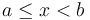$a \leq x < b$. It is often useful to express problems, algorithms, data structures, and library functions in terms of right half-open intervals of integers; a segment of an array is then specified by giving the left endpoint, that is, the index to the first element in the segment, and the right endpoint, which is the element just after the last element in the segment. For example, in C++, to sort a segment of an array A from the third element to the sixth element, inclusive, the code is std::sort(A+3, A+7);. The STL containers std::vector, std::list, std::deque, std::set, and std::map all have two member functions, begin() and end(), where the latter returns an iterator, not to the actual last element in the container, but to an imaginary element "just past the end".
• The interval$[a,b)$ contains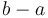$b-a$ elements exactly (rather than$b-a+1$ or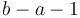$b-a-1$).
• The interval of length$n$ starting at$i$ can simply be denoted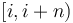$[i,i+n)$, rather than the more cumbersome$[i,i+n-1]$.
• The disjoint union of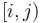$[i,j)$ and$[j,k)$ is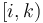$[i,k)$; and likewise any right half-open interval$[a,b)$ may be divided by intermediate values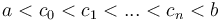$a < c_0 < c_1 < ... < c_n < b$ into a disjoint union of right half-open intervals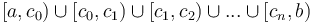$[a,c_0) \cup [c_0,c_1) \cup [c_1,c_2) \cup ... \cup [c_n, b)$; i.e., endpoints telescope nicely with half-open intervals, whereas they do not with fully open or fully closed intervals.